Apr 6, 2013

100 sided die probability problem

Source: Jane Street Capital Interview (Quantnet)

Problem:
You are given a 100 sided die. After you roll once, you can choose to either get paid the dollar amount of that roll OR pay one dollar for one more roll. What is the expected value of the game?
(There is no limit on the number of rolls.)

Update (22 June 2014):
Solution posted by me, Felix, JDGM, Sebastian, Fu Shi and Dumb Phoenix in comments!

1.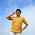If expected value of the game = E
At any turn, we roll the dice again we get a number less than [E-1]
[x] denotes the greatest integer less than or equal to x.

Probability of getting a number greater than [E-1] = (1 - [E-1])/100
Given that we rolled greater than [E-1], expected value of the prize = ([E]+100)/2

Expected number of rolls until we take the prize = 1/Probability of winning

So, we have to pay these many dollars until we win. Expected value of winning is:
(expected value of prize given that we win - expected number of rolls)

This expression should be equal to E.

Either I did something wrong up there, or I'm solving the equation wrong. I got E=102, which does not make sense. :-|

1.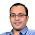Lets assume that expected value of the game = E
At any turn, we roll the dice again we get a number less than E-1

Assuming that the die gives a uniform value in [0,100]

E = (100-E-1)/100*((100+E-1)/2) + (E-1)*((E-1))/100
E = (101-E)*(99+E)/200 + (E-1)^2/100
200E = E^2-4E+2 + 9999 + 2E
E^2-202E+10001=0

Solving for E, and E<100,
we get, E=86.85

2.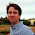The winning strategy is to play until you hit 87 or higher.
The expected value when you do is 93.5 (mean of 87 to 100).
The expectation of the needed number of rolls is 100 / 14, which means the end gain expectation is
E = 93.5 - 100 / 14
E = 1209 / 14
E ~= 86.357

(or plus 1 if the initial roll is free)

1.This is awesome. Thanks

3."If expected value of the game = E At any turn, we roll the dice again we get a number less than [E-1]"
---- This is not correct because interpretation of Expected Value is not correct.

1.Thanks. I was doing the same mistake.

4.Expected value of a roll is 50.5
Expected value of a roll after the first roll is 49.5 (since we need to pay 1)
The player stops if he gets any value >49.5, and continues if he gets a value <49.5
For the values 1 to 49, we can substitute with the expected value of 49.5
So expected value of the game = [49*49.5 + 50+51+...+100]/100 = 62.505

1."Expected value of a roll is 50.5
Expected value of a roll after the first roll is 49.5 (since we need to pay 1)" Thats wrong. You do not have to stop. The optionality gives a higher value to each roll

5.Félix has it.

I simmed this with 100 players each using a different "Keep playing until I roll X or greater" strategy (code here, put it in a compiler here), and then thought about the "why?".

Here's my process for actually proving it:

1. There are 2¹⁰⁰ possible strategies for a round: "If I get a {1, 2, …, 100}, I will {keep playing, stop playing}".

2. Any strategy which keeps playing on a certain roll, but stops playing on a lower roll than that (e.g. "If I get a 50 I will stop playing, if I get a 70 I will keep playing") is strictly inferior to the same strategy with those values switched.

3. Therefore, the only strategy for a round is "If I get X or higher I will stop, otherwise I will keep playing".

4. Round strategy does not change round to round as everything is the same except the amount of money in your "wallet", which has no effect on the round being played.

5. Therefore, the only strategy for the whole game is "If I get X or higher I will stop, otherwise I will keep playing".

6. The expected return for that strategy is:

Σ(n=1 to infinity):
P(roll≥X on nᵗʰ round) * Predicted \$ win
- P(roll<X on nᵗʰ round * \$1

It's a geometric series:

Σ(n=1 to infinity):
((X-1)/100)ⁿ⁻¹((101-X)/100) * (100+X)/2
- ((X-1)/100)ⁿ * 1

Simplifying and maximising for 0 < X <= 100, I get X = 101-10√2) ≈ 86.85786.

That's not quite what Félix got with his method. But, erm... ah well. The answer is still 87.

1.Oh, I have just seen why there was an apparent discrepancy with Félix's numbers...

I finished at finding the exact value of X in the strategy for a continuous "100-sided" dice, instead of converting it to the discrete case (which gives X=87) and plugging that back into the series to calculate the expected value of the game i.e. the actual answer we were looking for. Doing that gives me 1223/14, which is the same as Félix when you add the extra 1 for getting a free first roll.

The answer is 1223/14.

2.Bang on. Thanks

3.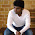@JDGM , I have a doubt with your 4th point : " Round strategy does not change round to round as everything is the same except the amount of money in your "wallet", which has no effect on the round being played"

A person playing the game wants to take home more money than what he brought,why will someone play more than 51 games as he already lost 50 bucks and the expected profit is (50.5-50) = 0.5.I think the optimal strategy for stopping the game changes for each round,the strategy should be calculated by dynamic approach (working backward from 51st round).....

Correct me if i'm wrong !!!! Am i missing something very basic ??

6.Answer: 1223/14 ~ 87,36

Defining the problem:

The average value of the entire game obviously depends on the strategy used. We can't include *every* possible scenario, because infinitely many of them would be losing scenarios (e.g. continuing to play even after you get 100 and infinitely many other silly scenarios). So we must choose a strategy that determines when to stop playing, and it must be the *optimal* strategy (i.e. resulting in the maximum average value) for there to be only one final answer to the problem.

If we decide to stop as soon as we get the number K or above, the average winning number is A = (100+K)/2. The average net result is A minus the number of rolls we pay for. The chance of succeding in the first roll is P(X>=K) and the chance of losing the first roll and succeding on the second is P(X=K) and so on...

The average value of the game is:

P(X>=K)*A + P(X=K)*(A-1) + P(X=K)*(A-2) + ...

This looks messy, so let's define p = P(X=K) = 1-p, so we get:

(1-p)*A + p*(1-p)*(A-1) + p^2*(1-p)*(A-2) + ...

or

(1-p) * (A + p*(A-1) + p^2*(A-2) + ...)

or

(1-p) * (A*p^0 + A*p^1 + A*p^2 + ... - 1*p - 2*p^2 - 3*p^3)

or

(1-p) * ( A*(p^0 + p^1 + p^2 + ...) - (p + 2*p^2 + 3*p^3 + ...) )

This still looks messy, but fortunately we can reduce the two infinite series:

(p^0 + p^1 + p^2 + ...) converges to 1/(1-p)
(p + 2*p^2 + 3*p^3 + ...) converges to p/(1-p)^2

so we get:

(1-p) * ( A*(1/(1-p)) - p/(1-p)^2 )

or

A - p/(1-p) or if you like: A - P(X=K)

Now that looks better! Plugging various numbers into this formula shows that 87 is indeed the optimal number, giving us the expected value:

93.5 - 86/100 / (14/100) = 93.5 - 86/14 = 2618/14 -86/14 = 2536/14 = 87,36

This calculation is much easier to do numerically in a spreadsheet with the following columns:

Roll no. | Money spent | P(win) | P(lose) | Contribution to expected value
1 | 0 | 0.14 | 0.86 | P(win)*(93.5 - Money spent) = 0.14*93.5
2 | 1 | 0.86*0.14~0.12 | 0.74 | P(win)*(93.5 - Money spent) = 0.12*92.5
3 | 2 | 0.74*0.12~0.104 | 0.6336 | P(win)*(93.5 - Money spent) = 0.104*91.5

Here P(win) and P(lose) are the probabilities of losing N-1 rolls followed by winning/losing the Nth roll. Do this for about 80-100 rows and calculate the sum of the "contributions" row.

7.If there were no provisions for playing again, one would have an expectation of 50.5 from the roll. Since you have to pay 1 dollar to roll again, your expected winning from any subsequent roll is 50.5-1 or 49.5. So only if you have rolled a number less than 49.5 would you be willing to roll again. This means that if you get a number from 1 to 49 (probability 0.49) you would roll again, but if you got a number from 50 to 100 (probability 0.51) you wouldn't roll again.

Let the expected winnings from the game be X.

E(X) = 0.49 * (Expected value from playing the game again) + 0.51 * 75
E(X) = 0.49*(-1 +E(X)) + 0.51*75

Solving, we get,
E(X) ~ 74.02

I'd be very thankful is someone could point out where I'm going wrong.

1.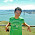your stopping rule is not optimal.

8.We can agree that stopping rule is to hit above some threshold m.
Let N = total number played, then N is geometrically distributed with p = (100 - m)/100.
Let T(m, N) = total earning after N plays with stopping threshold m (this means we hit >= m for all first N - 1 plays).
Denote T(m, N) as T whenever unambiguous.
Then

E(T) = E(E(T|N)) = E[(100 + (m+1))/2 - (N - 1)] = (101+m)/2 - E(N) + 1 = (101+m)/2 - 100/(100-m) + 1 = f(m)

set f'(m) = 1/2 - 100/(100 - m)^2 to 0 to get m = 100 - sqrt(200), check floor(m) and ceil(m) to obtain the optimal stopping thresh = 86, so you stop if you hit >86. Plug this into the expectation to get the answer.

1.Thanks. Interesting solution

9.I'm getting the value of the game to be 87.35
And the strategy to stop when you get more than 86.35

Here's a quick simulation in Excel for this:

1.10.First one needs to figure out a strategy to maximize his gain the game. This strategy will be : "play until you get equal to or greater than the expected value of the game."
Now, only thing remains is to actually find the expected value of the game.
So, let's say the expected value is K.
Then expected revenue (excluding the cost of playing) will be (K+100)/2. This is very simple.
Now, we need to figure out the cost of playing, that means the expected number of turns before the first success multiplied by cost of playing one turn. Now since we will succeed once we get any number greater or equal to K, probability of success is (100-(K-1))/100 .
So, expected value (K) = ((100+K)/2) - ((100-(K-1))/100) .
This will give you a quadratic equation, solving which you will get K=114.65 and K=86.35 . Obviously 114.65 is not the answer. So, the answer is greatest integer above 86.35 ie. 87

Fraction Brainteaser

Source: Sent to me by Gaurav Sinha Problem: Siddhant writes a Maths test and correctly answers 5 out of 6 Arithmetic questions and 20...